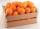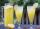# Video game

Nicole is playing a video game where each round lasts 7/12 of an hour. She has scheduled 3 3/4 hours to play the game. How many rounds can Nicole play?

Result

n =  6

#### Solution:Leave us a comment of this math problem and its solution (i.e. if it is still somewhat unclear...):Be the first to comment!#### Following knowledge from mathematics are needed to solve this word math problem:

Need help calculate sum, simplify or multiply fractions? Try our fraction calculator.

## Next similar math problems:

1. Pizza 5You have 2/4 of a pizza and you want to share it equally between 2 people how much pizza does each person get?
2. RoundingThe following numbers round to the thousandth:
3. Round it0.728 round to units, tenths, hundredths.
4. Crates 2One crate will hold 50 oranges. If Bob needs to ship 932 oranges, how many crates will he need?How many 1/4 cup servings are in 2 and 1/3 cups of lemonade?
6. Lengths of the poolMiguel swam 6 lengths of the pool. Mat swam 3 times as far as Miguel. Lionel swam 1/3 as far as Miguel. How many lengths did mat swim?
7. Fraction to decimalWrite the fraction 3/22 as a decimal.
8. ZdeněkZdeněk picked up 15 l of water from a 100-liter full-water barrel. Write a fraction of what part of Zdeněk's water he picked.
9. Fraction and a decimalWrite as a fraction and a decimal. One and two plus three and five hundredths
10. Write 2Write 791 thousandths as fraction in expanded form.
11. Fractions 4How many 2/3s are in 6?
12. Cupcakes 2Susi has 25 cupcakes. She gives 4/5. How much does she have left?
13. Mixed2improperWrite the mixed number as an improper fraction. 166 2/3
14. Pizza 4Marcus ate half pizza on monday night. He than ate one third of the remaining pizza on Tuesday. Which of the following expressions show how much pizza marcus ate in total?
15. TeacherTeacher Rem bought 360 pieces of cupcakes for the outreach program of their school. 5/9 of the cupcakes were chocolate flavor and 1/4 wete pandan flavor and the rest were a vanilla flavor. How much more pandan flavor cupcakes than vanilla flavor?
16. Product of two fractionsProduct of two fractions is 9 3/5 . If one of the fraction is 9 3/7. Find the other fraction.
17. Passenger boatTwo-fifths of the passengers in the passenger boat were boys. 1/3 of them were girls and the rest were adult. If there were 60 passengers in the boat, how many more boys than adult were there?